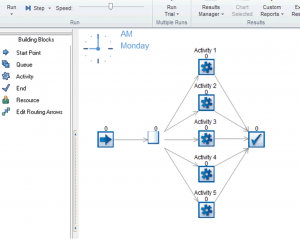Written by Charlie on Friday September 6th 2013 in SIMUL8 Tips, Technical Corner

## SIMUL8 Tip – How to set up a Speed List

Setting up variables that reference simulation objects is essential when writing complex Visual Logic for SIMUL8.

However, when your simulation employs a large number of variable names, (e.g. when using Component objects or looping through a long spreadsheet of names) this can impact the speed of your simulation from the large number of objects needing looked up by text.

Speed Lists can address this problem by creating one-off automatically generated reference codes for each of your object variables. Let’s explore how to do this.

For simplicity’s sake, let’s look at an example using a basic simulation with 5 Activities.As can be seen, we have a basic simulation with a Start Point, a Queue, 5 Activities and an End.

We then need to create a simple spreadsheet (we’ll call it ‘ssSpeedList’) of 2 columns by 5 rows which will contain the automatically generated references to correspond to these 5 activities, like so:NB that the ‘Ref Codes’ column is left blank. This is so our reference codes can now be generated automatically, using Visual Logic.

We do this by accessing SIMUL8’s Visual Logic tab. Use the following command: